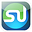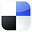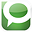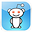# and Then Remove Them

If you are an Internet marketer, you already know the importance of list management skills. Nothing beats Excel as a platform to manipulate the data in your lists.

Here is a quick lesson on how to find matching records in 2 lists in just 2 steps using VLOOKUP. We will then show you how to quickly remove those matches from either of these lists, leaving you with the original list with all records that have no matches in the other list.

Finding Matches in 2 Lists Using VLOOKUP

Step 1 – Arrange the Lists Properly and Perform a VLOOKUP

Here are the 2 lists. Arrange them so that the larger list is on the left:

Now, perform your VLOOKUP. Type the formula shown below into cell D2 and copy it down to cell D9. The results of the VLOOKUP appear in cells D2 to D9. An explanation of this VLOOKUP is provided next.

Click on the Image To See Larger Version

Here is an explanation of each argument of the VLOOKUP function in this case:

Column D contains the results of the VLOOKUP. The actual contents of the cells in this column are the VLOOKUP formulas, not the values showing in the cells. We need to work with only the values that appear and not the formulas.

Step 2 - Copy and Sort the Values from VLOOKUP

To do this, copy the contents of the column and then paste only their values using Paste / Paste Special / Values as shown here:

Click on the Image To See Larger Version

Now that we have a column of values and not formulas, we can sort the column with the ordinary Sort function under the Data tab as follows:

Click on the Image To See Larger Version

The Matches in Both Lists

Here is the result of the Sort. Highlighted in yellow are the matches from both lists:

Removing the Matches From List 1.

Paste the matches at the bottom of List 1:

Sort List 1 to get this result:

Use an If-Then-Else statement to print any cells that do not have matches above or below them. If a cell above or below matches that cell, only a blank space (“ “) will be printed. The formula shown in cell Q4 can be copied in cells Q2 to Q13 to get the following result:

Click On Image To See Larger Version

The contents of the cells in column Q are actually formulas, not the numbers that appear in the cells that are the result of the calculation. We need to copy only those numerical values into a column so we can perform data operations on them.

Do this the same way it was done above. Copy the contents of cells Q2 to Q13 and paste them into a new column using Paste Special / Values to get the following result:

Click On Image To See Larger Version

Sorting these will give you the final List 1 (highlighted in yellow below) with all matches from List 2 removed:

Click On Image To See Larger Version

You could easily run the same removal procedure on List 2 to remove the matches from that list as well. If you did that, you would have 2 lists that had no matching records.

If you would like to create a link to this blog article, here is the link to copy for your convenience: Using VLOOKUP In Excel To Find and Remove Matches in 2 ListsIf You Like This, Then Share It...Excel Master Series Blog Directory

Statistical Topics and Articles In Each Topic

• Histograms in Excel
• Bar Chart in Excel
• Combinations & Permutations in Excel
• Normal Distribution in Excel
• t-Distribution in Excel
• Binomial Distribution in Excel
• z-Tests in Excel
• t-Tests in Excel
• Hypothesis Tests of Proportion in Excel
• Chi-Square Independence Tests in Excel
• Chi-Square Goodness-Of-Fit Tests in Excel
• F Tests in Excel
• Correlation in Excel
• Pearson Correlation in Excel
• Spearman Correlation in Excel
• Confidence Intervals in Excel
• Simple Linear Regression in Excel
• Multiple Linear Regression in Excel
• Logistic Regression in Excel
• Single-Factor ANOVA in Excel
• Two-Factor ANOVA With Replication in Excel
• Two-Factor ANOVA Without Replication in Excel
• Creating Interactive Graphs of Statistical Distributions in Excel
• Solving Problems With Other Distributions in Excel
• Optimization With Excel Solver
• Chi-Square Population Variance Test in Excel
• Analyzing Data With Pivot Tables
• SEO Functions in Excel
• Time Series Analysis in Excel

1.2.